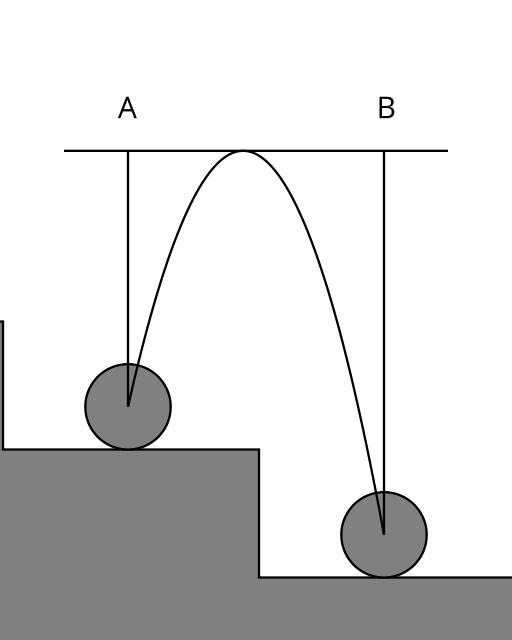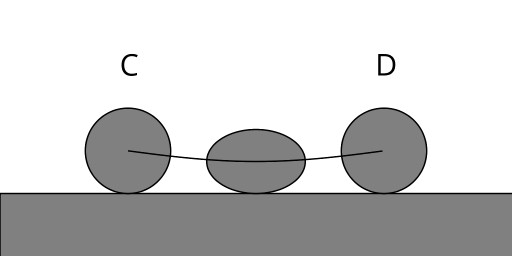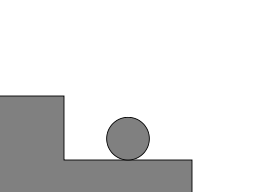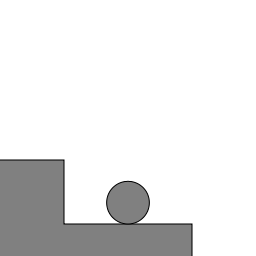# mathr / blog / #

## A ball bouncing down steps

The first part of the process is a ball in free flight from one step down to the next:Consider gravitational potential energy $$m g h$$ (where m is mass, g is the force of gravity, and h is the height) at the peak height $$H$$ of the flight path over steps of height $$S$$ relative to A and B:

\begin{aligned} E_A &= m g (H - S) \\ E_B &= m g H \end{aligned}

Consider kinetic energy $$\frac{1}{2} m v^2$$ at A and B:

\begin{aligned} E_A &= \frac{1}{2} m v_A^2 \\ E_B &= \frac{1}{2} m v_B^2 \end{aligned}

Some simple algebra gives:

\begin{aligned} v_A = \sqrt{ 2 g (H - S) } \\ v_B = \sqrt{ 2 g H } \end{aligned}

When in flight, the only force acting on the ball is gravity. Using Newton's law of motion relating force and acceleration $$F = m a$$ and integrating with the initial conditions at $$A$$ with $$t = 0$$ gives:

\begin{aligned}\frac{\partial}{\partial t}\frac{\partial}{\partial t} y & = -g \\ \frac{\partial}{\partial t} y &= v_A - g t \\ y &= r + v_A t - \frac{1}{2} g t^2 \end{aligned}

where $$y$$ is the height of the ball center and $$r$$ is the ball radius. Now we can find the duration $$T_f$$ of the flight, which ends when $$y(T_f) = y(0) - S$$:

\begin{aligned} r - S &= r + v_A T_f - \frac{1}{2} g T_f^2 \\ \frac{1}{2} g T_f^2 - v_A T_f - S &= 0 \\ T_f = \frac{v_A + \sqrt{ v_A^2 + 2 g S } }{ g } \end{aligned}

The second part of the process is a compressive bounce when the ball reaches the lower step:Modelling the bounce by a damped harmonic oscillator gives an equation of motion, where $$d$$ is the maximal squashing of the ball:

$y = r \left( 1 - d \sin (\beta t) e^{-\gamma t} \right)$

Here $$\beta$$ determines the speed of the bounce and $$\gamma$$ determines the energy lost during the bounce. Using the boundary conditions $$v_C = v_B$$ and $$v_D = v_A$$ for a smooth transition from free flight to bouncing, and setting $$T_b$$ to be the duration of the bounce (time of first return to initial $$y$$ value), we can differentiate the equation of motion and solve for the unknowns:

\begin{aligned} \frac{\partial}{\partial t} y &= r e^{-\gamma t} \left( \gamma \sin(\beta t) - d \beta \cos(\beta t) \right) \\ v_B &= r d \beta \\ \beta &= \frac{v_B}{r d} \\ T_b &= \frac{\pi}{\beta} \\ v_A &= r e^{-\gamma T_b} d \beta \\ \gamma &= -\frac{\log \frac{v_A}{r d \beta}}{T_b} \end{aligned}

The time taken for the whole loop is $$T = T_f + T_b$$. Assuming the ball is initially spherical, and that volume remains constant, we can compute the squashing factor using the property of an ellipsoid that $$V = k r_x r_y r_z$$ assuming that $$r_x = r_z$$:

\begin{aligned} r_y &= y \\ r_x &= \sqrt{\frac{r^3}{y}} \end{aligned}

Implementing all these equations in the Haskell programming language using the Diagrams library for visualization gives some cute animated GIFs: